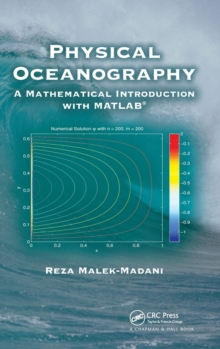Supporting your high street Find out how »
• My Account# Physical Oceanography : A Mathematical Introduction with MATLAB Hardback

#### Description

Accessible to advanced undergraduate students, Physical Oceanography: A Mathematical Introduction with MATLAB (R) demonstrates how to use the basic tenets of multivariate calculus to derive the governing equations of fluid dynamics in a rotating frame.

It also explains how to use linear algebra and partial differential equations (PDEs) to solve basic initial-boundary value problems that have become the hallmark of physical oceanography.

The book makes the most of MATLAB's matrix algebraic functions, differential equation solvers, and visualization capabilities. Focusing on the interplay between applied mathematics and geophysical fluid dynamics, the text presents fundamental analytical and computational tools necessary for modeling ocean currents.

In physical oceanography, the fluid flows of interest occur on a planet that rotates; this rotation can balance the forces acting on the fluid particles in such a delicate fashion to produce exquisite phenomena, such as the Gulf Stream, the Jet Stream, and internal waves.

It is precisely because of the role that rotation plays in oceanography that the field is fundamentally different from the rectilinear fluid flows typically observed and measured in laboratories.

Much of this text discusses how the existence of the Gulf Stream can be explained by the proper balance among the Coriolis force, wind stress, and molecular frictional forces. Through the use of MATLAB, the author takes a fresh look at advanced topics and fundamental problems that define physical oceanography today.

The projects in each chapter incorporate a significant component of MATLAB programming.

These projects can be used as capstone projects or honors theses for students inclined to pursue a special project in applied mathematics.

#### Information

• Format: Hardback
• Pages: 456 pages, 85 Illustrations, black and white
• Publisher: Taylor & Francis Inc
• Publication Date:
• Category: Oceanography (seas)
• ISBN: 9781584888307

£75.00

£66.19